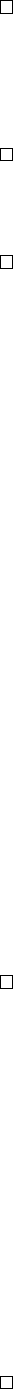3Integration

II Probability and Measure3.3 New measures from old
We have previously considered several ways of constructing measures from old
ones, such as the image measure. We are now going to study a few more ways of
constructing new measures, and see how integrals behave when we do these.
Definition
(Restriction of measure space)
.
Let (
E, E, µ
) be a measure space,
and let A E. The restriction of the measure space to A is (A, E
A
, µ
A
), where
E
A
= {B E : B A},
and µ
A
is the restriction of µ to E
A
, i.e.
µ
A
(B) = µ(B)
for all B E
A
.
It is easy to check the following:
Lemma.
For (
E, E, µ
) a measure space and
A E
, the restriction to
A
is a
measure space.
Proposition.
Let (
E, E, µ
) and (
F, F, µ
0
) be measure spaces and
A E
. Let
f : E F be a measurable function. Then f|
A
is E
A
-measurable.
Proof. Let B F. Then
(f|
A
)
1
(B) = f
1
(B) A E
A
.
Similarly, we have
Proposition.
If
f
is integrable, then
f|
A
is
µ
A
-integrable and
µ
A
(
f|
A
) =
µ(f1
A
).
Note that means we have
µ(f1
A
) =
Z
E
f1
A
dµ =
Z
A
f dµ
A
.
Usually, we are lazy and just write
µ(f1
A
) =
Z
A
f dµ.
In the particular case of Lebesgue integration, if
A
is an interval with left and
right end points
a, b
(i.e. it can be open, closed, half open or half closed), then
we write
Z
A
f dµ =
Z
b
a
f(x) dx.
There is another construction we would be interested in.
Definition
(Pushforward/image of measure)
.
Let (
E, E
) and (
G, G
) be measure
spaces, and
f
:
E G
a measurable function. If
µ
is a measure on (
E, E
), then
ν = µ f
1
is a measure on (G, G), known as the pushforward or image measure.
We have already seen this before, but we can apply this to integration as
follows:
Proposition. If g is a non-negative measurable function on G, then
ν(g) = µ(g f).
Proof. Exercise using the monotone class theorem (see example sheet).
Finally, we can specify a measure by specifying a density.
Definition
(Density)
.
Let (
E, E, µ
) be a measure space, and
f
be a non-negative
measurable function. We define
ν(A) = µ(f1
A
).
Then ν is a measure on (E, E).
Proposition. The ν defined above is indeed a measure.
Proof.
(i) ν(φ) = µ(f1
) = µ(0) = 0.
(ii) If (A
n
) is a disjoint sequence in E, then
ν
[
A
n
= µ(f1
S
A
n
) = µ
f
X
1
A
n
=
X
µ (f1
A
n
) =
X
ν(f).
Definition
(Density)
.
Let
X
be a random variable. We say
X
has a density if
its law
µ
X
has a density with respect to the Lebesgue measure. In other words,
there exists f
X
non-negative measurable so that
µ
X
(A) = P[X A] =
Z
A
f
X
(x) dx.
In this case, for any non-negative measurable function, for any non-negative
measurable g, we have that
E[g(X)] =
Z
R
g(x)f
X
(x) dx.